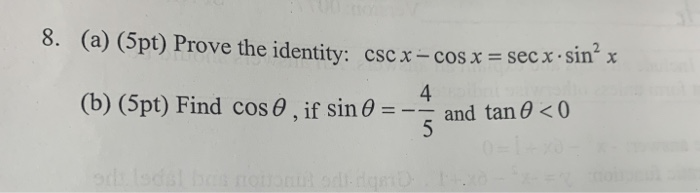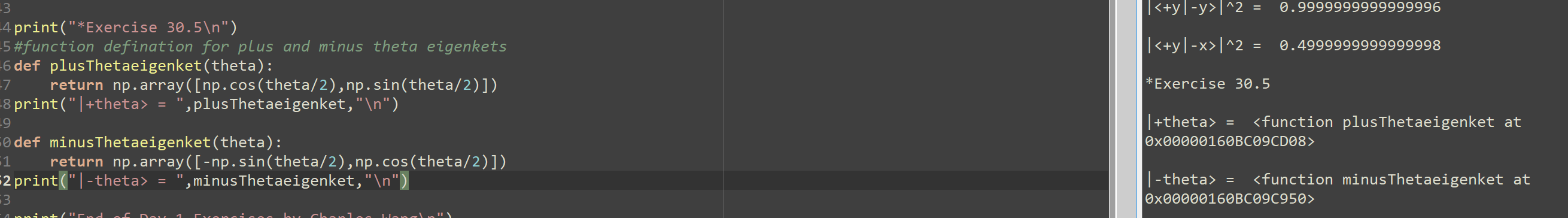# sin^2 theta + 2 cos theta -1/ (sin^2 theta +3 cos theta-3= cos^2 theta+cos theta/ (-sin^2 theta) Prove the identity related homework questions

• #### It’s review question, I need this as soon as possible. Thank you 3) For thè diferential equation: (a) The point zo =-1 is an ordinary point. Compute the recursion formula for the coefficients of...It’s review question, I need this as soon as possible. Thank you 3) For thè diferential equation: (a) The point zo =-1 is an ordinary point. Compute the recursion formula for the coefficients of the power series solution centered at zo- -1 and use it to compute the first three nonzero terms of the power series when -1)-s and v(-1)-0....

• #### Dont copié formé thé book oh ya dont copié formé thé book cause you Oiil inde up being triste soi remembré not toi copié frome thé book oh ya

Dont copié formé thé book oh ya dont copié formé thé book cause you Oiil inde up being triste soi remembré not toi copié frome thé book oh ya!translation in english please!

• #### sin^2 theta + 2 cos theta -1/ (sin^2 theta +3 cos theta-3= cos^2 theta+cos theta/ (-sin^2 theta) Prove the identity

sin^2 theta + 2 cos theta -1/ (sin^2 theta +3 cos theta-3= cos^2 theta+cos theta/ (-sin^2 theta)Prove the identity.

• #### DSuppose \$39oo is deposited in a savings account that increases exponentially.Detamine thě APv if the acount...DSuppose \$39oo is deposited in a savings account that increases exponentially.Detamine thě APv if the acount increases to \$t020 in 4 years. Ass ume tne interest Vale remains Constant and no additional deposits or Withdrawals are made. (a.) Let pbe the APY. Note tnat if tme inital balaqe is yo, ne year later tne balane is %more. P- 3 (Tpe...

• #### Is sin theta minus cos theta = root 2 Sin Theta then prove that sin theta + cos theta is equals to root sin theta?

If #sintheta-costheta=sqrt(2)sintheta#, then prove that, #sintheta+costheta=-sqrt(2)costheta#

• #### 8. (a) (5pt) Prove the identity: cscx -cos x=sec x . sin'x 4 and tan θ<0 (b) (5pt) Find cos θ , if sin θ 8. (a) (5pt) Prove the identity: cscx -cos x=sec x . sin'x 4 and tan θ8. (a) (5pt) Prove the identity: cscx -cos x=sec x . sin'x 4 and tan θ<0 (b) (5pt) Find cos θ , if sin θ 8. (a) (5pt) Prove the identity: cscx -cos x=sec x . sin'x 4 and tan θ

• #### prove the identity : cos^4 theta- sin ^4 thetaover sin squared theta cos squared theta =cot squared theta - tan squared theta

prove the identity : cos^4 theta- sin ^4 thetaover sin squared theta cos squared theta =cot squared theta - tan squared theta

• #### def plusThetaeigenket(theta): return np.array([np.cos(theta/2),np.sin(theta/2)]) print("|+theta> = ",plusThetaeigenket,"\n") def minusThetaeigenket(theta): return np.array([-np.sin(theta/2),np.cos(theta/2)]) print("|-theta> = ",minusThetaeigenket,"\def plusThetaeigenket(theta): return np.array([np.cos(theta/2),np.sin(theta/2)]) print("|+theta> = ",plusThetaeigenket,"\n") def minusThetaeigenket(theta): return np.array([-np.sin(theta/2),np.cos(theta/2)]) print("|-theta> = ",minusThetaeigenket,"\n") Can you please help to solve where this python code went wrong for display the correct eigen ket value for theta? Thank you |<+y|-y>l^2 = 0.9999999999999996 ||<+y|-x>l^2 = 0.4999999999999998 -4 print("*Exercise 30.5\n"), -5 #function defination for plus and minus theta eigenkets -6 def plusThetaeigenket(theta): +7 return...

• #### Prove that Sin(theta)times Cos(theta) divided by Cos^2(theta)-Sin^2(theta)=Tan(theta) divided by 1-Tan(theta) Show step by step how to change the left to equal the right side of equasion

Prove that Sin(theta)times Cos(theta) divided by Cos^2(theta)-Sin^2(theta)=Tan(theta) divided by 1-Tan(theta) Show step by step how to change the left to equal the right side of equasion

• #### 1)Use the definitions sin0(theta) = y/r, cos0(theta) = x/r and/or tan0(theta) = y/x to prove that cot0(theta) = 1/tan0(theta) 2)For all 0(theta) prove: a)cos(t) = 1/sec0(theta) b

1)Use the definitions sin0(theta) = y/r, cos0(theta) = x/r and/or tan0(theta) = y/x to prove that cot0(theta) = 1/tan0(theta) 2)For all 0(theta) prove: a)cos(t) = 1/sec0(theta) b. sec(t) = 1/cos0(theta)

• #### 1)Use the definitions sin0(theta) = y/r, cos0(theta) = x/r and/or tan0(theta) = y/x to prove that cot0(theta) = 1/tan0(theta) 2)For all 0(theta) prove: a)cos(t) = 1/sec0(theta) b

1)Use the definitions sin0(theta) = y/r, cos0(theta) = x/r and/or tan0(theta) = y/x to prove that cot0(theta) = 1/tan0(theta) 2)For all 0(theta) prove: a)cos(t) = 1/sec0(theta) b. sec(t) = 1/cos0(theta)

• #### Prove that Cot(theta)/1-Tan(theta) + Tan(theta)/1-Cot(theta) = 1+Tan(theta)+Cot(theta) must show step by step I have tried a couple of steps by changing Cot to 1-1/Cot(theta) and 1-Tan(theta) 1-1/Cot (theta)

Prove that Cot(theta)/1-Tan(theta) + Tan(theta)/1-Cot(theta) = 1+Tan(theta)+Cot(theta) must show step by step I have tried a couple of steps by changing Cot to 1-1/Cot(theta) and 1-Tan(theta) 1-1/Cot (theta)

• #### In studying the interference of light waves, the identity #sin(x)sin[(3/2)x]/sin[(1/2)x] = sin(x) + sin(2x) # is used. Prove this identity?

#sin(x)sin(3/2x)/sin(1/2x )= sin(x) + sin(2x)# Prove this identity

• #### Pove that 1+sin(theta)divided by 1-Sin(theta)- 1-Sin(theta) Divided by 1+Sin(theta)= 4Tan(theta)times Sec(theta) Show step by step how to change the left side of the equation to equal the right side of the equation

Pove that 1+sin(theta)divided by 1-Sin(theta)- 1-Sin(theta) Divided by 1+Sin(theta)= 4Tan(theta)times Sec(theta) Show step by step how to change the left side of the equation to equal the right side of the equation.

• #### If #Cos theta +sin theta = sqrt2 sin theta# then #sin theta - cos theta#?

Free Homework App